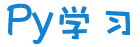注册    登录创作新主题

Python
 python开源   Django   Python   DjangoApp   pycharm
DATA
 docker   Elasticsearch

 问与答   闲聊   招聘   翻译   创业   分享发现   分享创造   求职   区块链   支付之战
aigc
 aigc   chatgpt
WEB开发
 linux   MongoDB   Redis   DATABASE   NGINX   其他Web框架   web工具   zookeeper   tornado   NoSql   Bootstrap   js   peewee   Git   bottle   IE   MQ   Jquery

Python88.com
 反馈   公告   社区推广

Py学习  »  Python

# HF.055｜科研绘图王炸组合ChatGPT+Python

Hydro90 • 5 月前 • 192 次点击01

（1）北京市各区县地图矢量数据（shp文件）。
（2）降雨质心（可理解为场次降雨某个时刻的降雨中心）坐标，对应降雨时刻与雨强大小，具体如下表所示：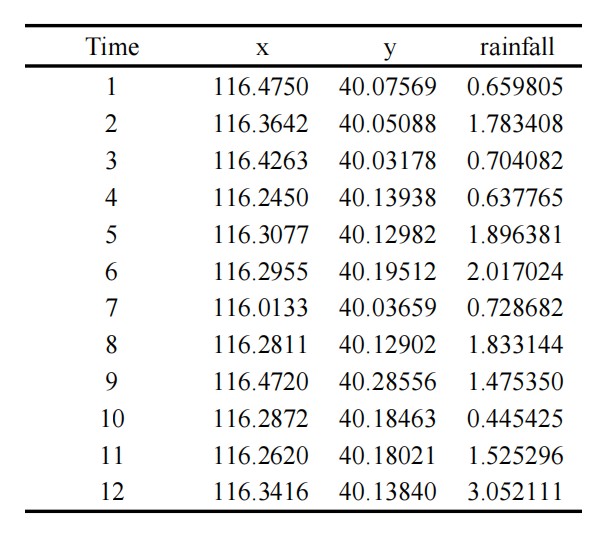02

“用python帮我绘制高级美观的气泡地图，在pycharm里运行显示，数据路径为C:\Users\86136\Desktop\Data.xlsx，数据格式为上文提到表格形式。”

“为了绘制高级美观的泡泡地图，我们可以使用Python中的一些库来实现，包括Pandas、Matplotlib和Seaborn，完整代码如下：”

``import pandas as pd``import matplotlib.pyplot as plt``import seaborn as sns``data = pd.read_excel('C:\\Users\\86136\\Desktop\\Data.xlsx')`` ``sns.scatterplot(x='x', y='y', data=data, hue='rainfall', size='rainfall', sizes=(50, 500))``plt.show()``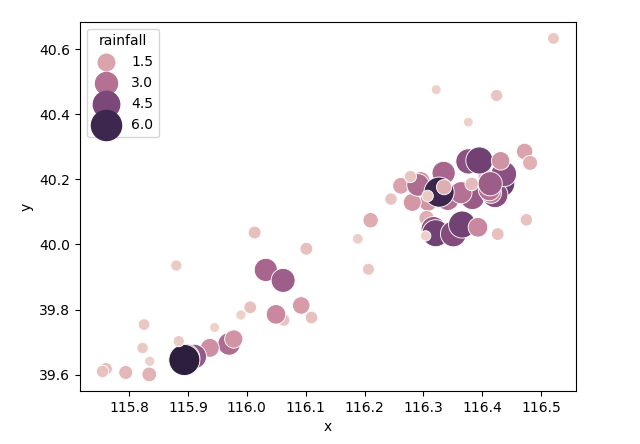03

“帮我优化代码，time值表示气泡图的颜色深浅，rainfall值表示气泡图大小。”

“在这个问题中，我们可以根据时间值为气泡地图设置颜色深浅，并将rainfall值用于确定泡泡图的大小。”

``import pandas as pd``import matplotlib.pyplot as plt``import seaborn as sns`` ``data = pd.read_excel('C:\\Users\\86136\\Desktop\\Data.xlsx')`` ``sns.scatterplot(x='x', y='y', data=data, hue='time', size='rainfall', sizes=(50, 500), palette='coolwarm')``plt.show()``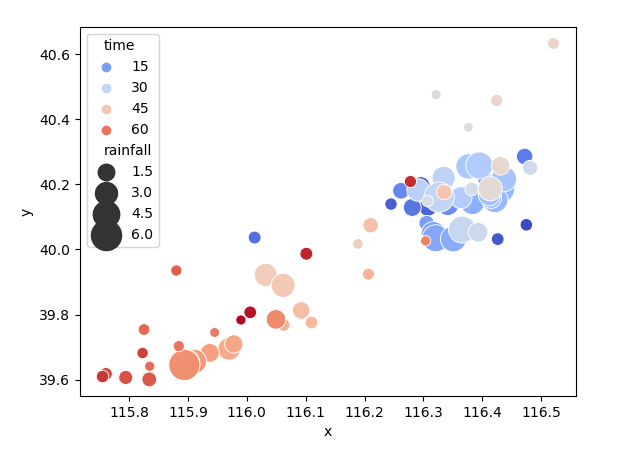“帮我优化代码，要求保持原有的气泡图大小颜色，然后绘制在我提供的shp文件范围中。”

“为了保持原有的气泡图大小和颜色，并将它们绘制在提供的shp文件范围内，我们可以使用geopandas库。首先，我们需要导入所需的库和数据。”

``import pandas as pd``import geopandas as gpd``from shapely.geometry import Point``import matplotlib.pyplot as plt``import seaborn as sns`` # 从Excel文件中读取数据``data = pd.read_excel('C:\\Users\\86136\\Desktop\\Data.xlsx')`` # 从shp文件中读取区县边界数据``shp_path = 'C:\\Users\\86136\\Desktop\\shp\\北京市区县.shp'``map_df = gpd.read_file(shp_path)`` # 将x、y坐标转换为Point对象``geometry = [Point(xy) for xy in zip(data['x'], data['y'])]`` # 创建GeoDataFrame``geo_data = gpd.GeoDataFrame(data, crs=map_df.crs, geometry=geometry)`` # 空间连接``merged = gpd.sjoin(map_df, geo_data, op='contains')`` # 绘制图表``fig, ax = plt.subplots(1, figsize=(10, 6))``sns.scatterplot(x='x', y='y', data=data, hue='time', size='rainfall', sizes=(50, 500), palette='coolwarm', alpha=0.7, legend=False, ax=ax)``merged.plot(facecolor='none', edgecolor='black', linewidth=0.5, ax=ax)``plt.show()``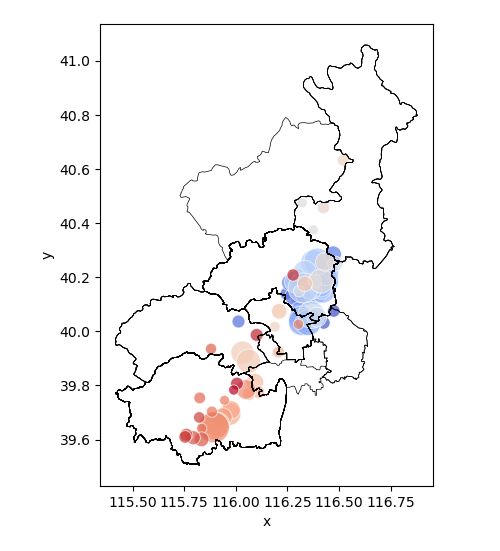04

“继续优化代码，要求按之前的要求添加图例。”

“为了按照之前的要求绘制气泡图，并在提供的shp文件范围内添加图例并完全显示，我们可以使用geopandas和Seaborn库。”
``import pandas as pd``import geopandas as gpd``from shapely.geometry import Point``import matplotlib.pyplot as plt``import seaborn as sns``plt.rcParams['font.family'] = 'Times New Roman'``# 从Excel文件中读取数据``data = pd.read_excel('C:\\Users\\86136\\Desktop\\Data.xlsx')``# 从shp文件中读取区县边界数据``shp_path = 'C:\\Users\\86136\\Desktop\\shp\\北京市区县.shp'``map_df = gpd.read_file(shp_path)``# 将x、y坐标转换为Point对象``geometry = [Point(xy) for xy in zip(data['x'], data['y'])]``# 创建GeoDataFrame``geo_data = gpd.GeoDataFrame(data, crs=map_df.crs, geometry=geometry)``# 空间连接``merged = gpd.sjoin(map_df, geo_data, op='contains')``# 绘制图表``fig, ax = plt.subplots(1, figsize=(96, 87))``sns.scatterplot(x='x', y='y', data=data, hue='Time', size='Rainfall', sizes=(50, 500), palette='coolwarm', alpha=0.7, legend='brief', ax=ax)``merged.plot(facecolor='none', edgecolor='black', linewidth=0.5, ax=ax)``ax.set_xlabel('Longitude', fontsize=14)``ax.set_ylabel('Latitude', fontsize=14)``legend = ax.legend(loc='upper left', prop={"family": "Times New Roman"})``# 调整图例框大小``ax.legend(handlelength=3, handleheight=1)``#ax.legend(frameon=False)``ax.legend(fontsize=13.6)``# 调整图例位置``ax.get_legend().set_bbox_to_anchor((0.25, 1))``plt.show()``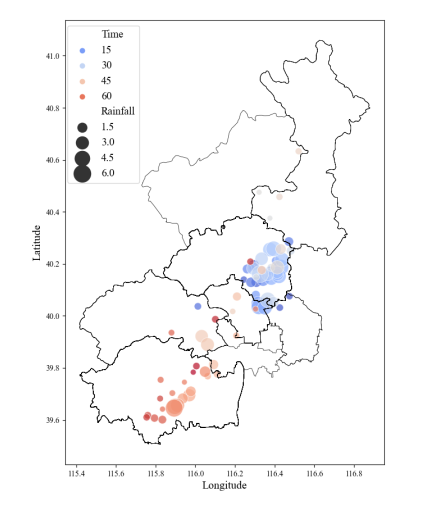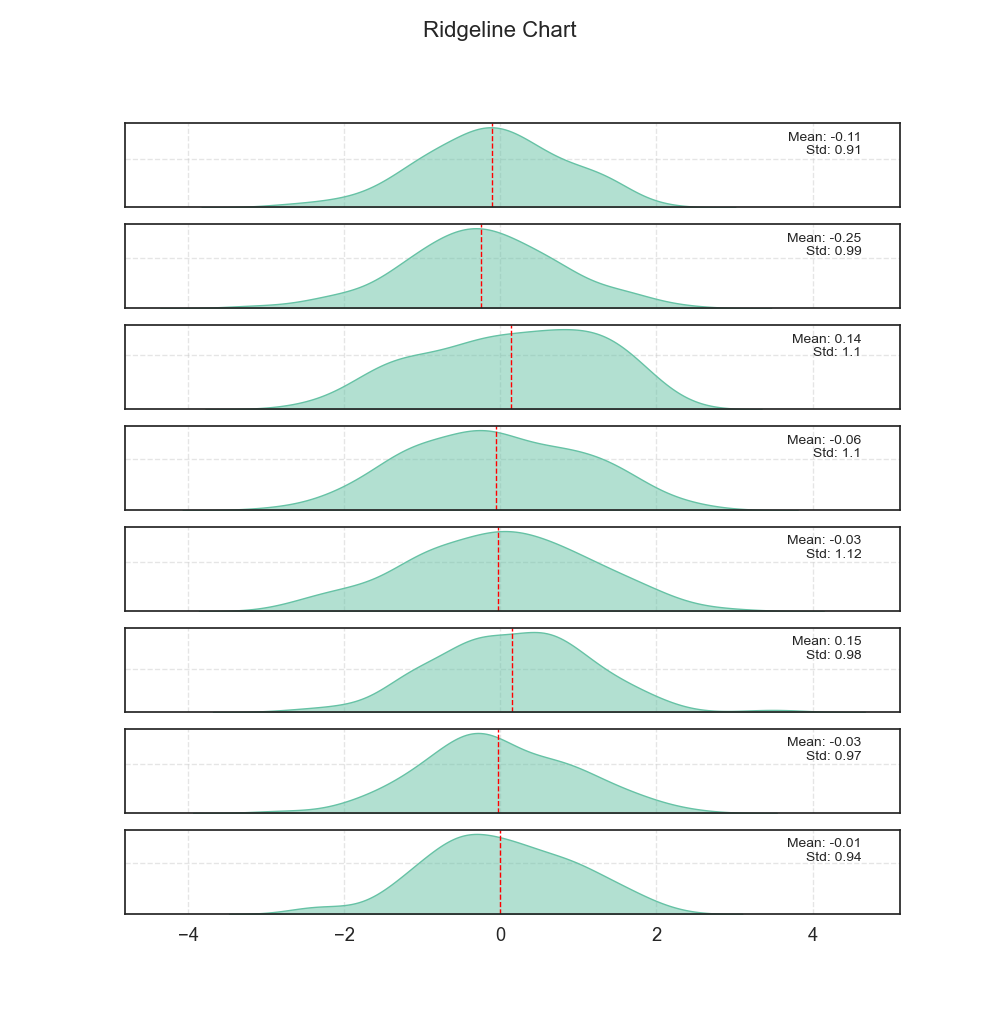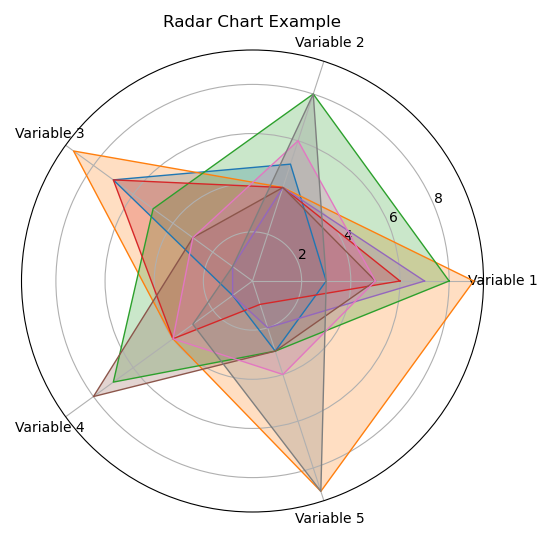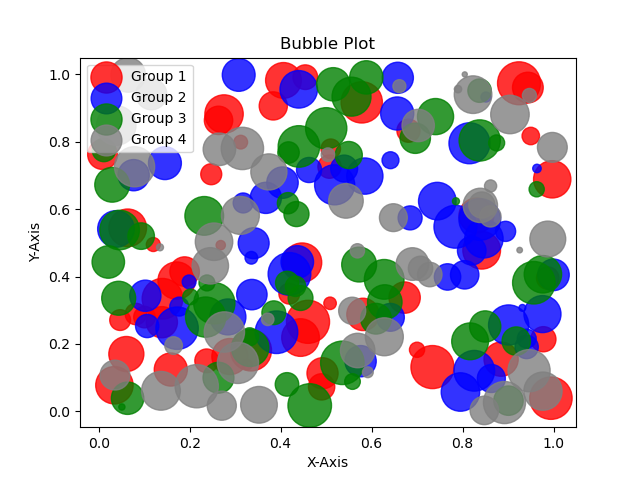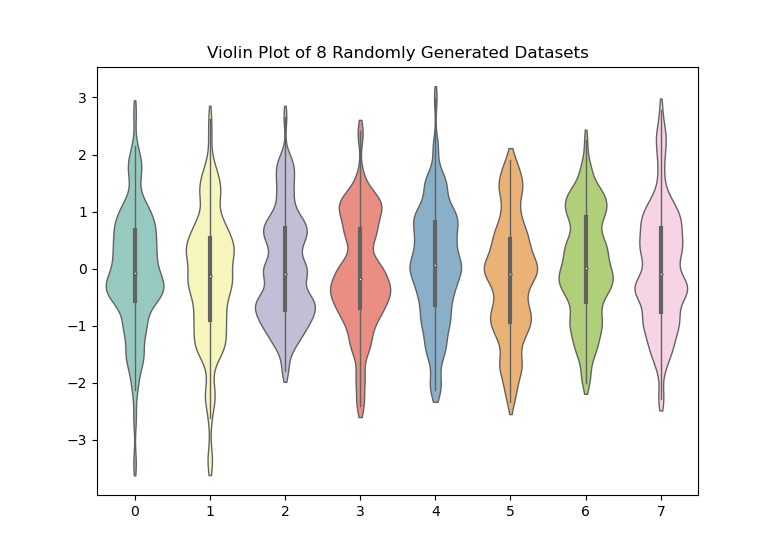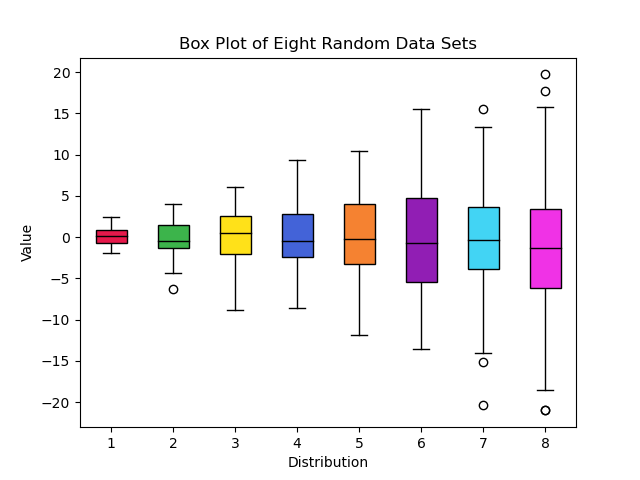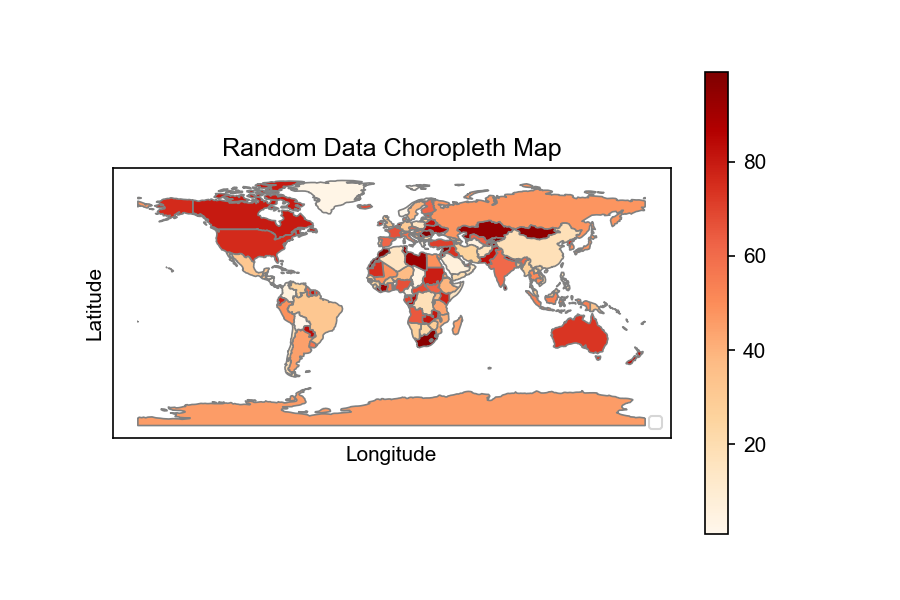05

1.在表述准确的前提下使用英文提问效果要好于中文提问；在提问最开始限制使用的编程语言和使用的具体绘图库会精确代码的方向；出错报错后可以通过继续向ChatGPT追问得到解决。

2.一般的提问格式可以按照：前提假设（使用何种编程语言）---数据样本（提供给ChatGPT的数据样本格式）---需求（需要何种类型的绘图）。

3.对于无代码基础者，建议遵循以下原则：对绘图所需数据进行充分预处理，便于ChatGPT识别；分多次提问，第一步识别数据，第二步绘制所需基本图形，第三步对基本图形进行调整，以减少报错及报错后的调试难度；有逻辑的表述自己的意图，比如说清楚具体需要绘制什么类型的图表。

1.常用绘图类型

The R Graph Gallery 网址 ：

https://r-graph-gallery.com/index.html

Data to Viz 网址 ：

https://www.data-to-viz.com/

2.ChatGPT 常用镜像网站汇总

https://github.com/LiLittleCat/awesome-free-chatgpt

3.Pycharm（专业版）ChatGPT插件

https://blog.csdn.net/weixin_57664381/article/details/129665074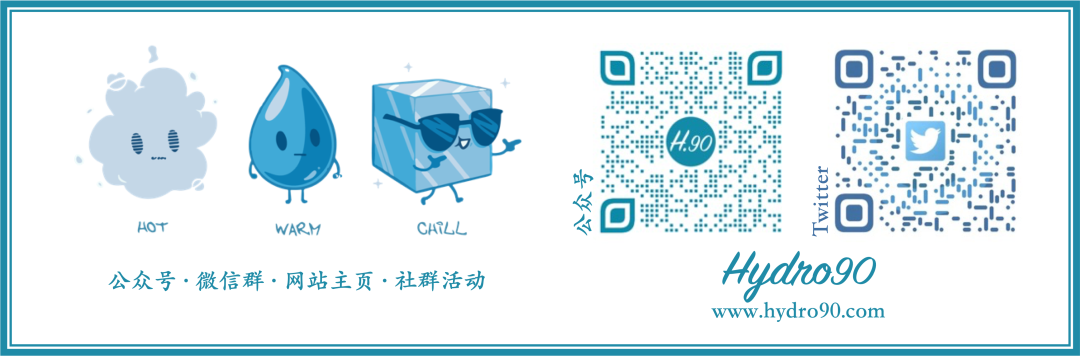Python社区是高质量的Python/Django开发社区